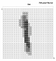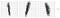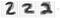# Understanding the MNIST and building classification model with MNIST and Fashion MNIST datasets

Level: Beginner

Python understanding: Intermediate

Knowledge of data science: Intermediate

Objective: Develop an intuition of multi-dimensional dataset.

Goal: A trained model which does Image Classification

IDE: To get started I will recommend using Jupyter notebook on Google Collaboration.

Regarded as the hello world of Deep Learning, this dataset exposes inspiring data scientists to the complexity which exist in the real world.

The intention is to share how I have learned to understand the world of multi-dimensional arrays.

So, here is the challenge when we look at the dataset, it looks like a floating-point number in arrays if you run the code X_train.

Visualising multiple dimensions of the dataset

These numbers relate to each image in the MNIST dataset, and that is what I’ll be looking into further.

The image that we see here is:

Although it doesn’t matter which image I select to examine further but to keep things intuitive, let's select the first image.

We are associating this pattern with the digit 1 because we have a history of association with numbers.

The algorithm doesn’t have any association with the mathematical notation of, 1 but we aim to teach the machine that all patterns which are closely related to the same number.

If a pattern is like a straight line in the image then learn to associate them as 1. There are several ways human may write 1 and the goal is to give the machine the ability to recognize these patterns.

The dataset has classes where similar images belong to the same class. You can see the example of the class labelled as 1.

We have to take a step back at this point to understand what is happening in the images above.

The images are a flattened form of scanned jpeg into 28 pixels in height and 28 pixels in width.

We know each image is made of 28 x 28 pixels and there is a distinct pattern on each grid so let’s answer the question of how do we teach the algorithm to learn the pattern?

To answer that we will examine each pixel. Each pixel has the intensity of a shade represented by the value between 0 and 1 which can be transformed to be between 0 and 255.

We know so far that there are images in MNIST dataset, this data was transformed to 28x28 pixel grid and each pixel have a value between either 0 to 1 or 0 to 255 and if we combine what we know so far, this is the kind of image we will get.

Now let’s, look at what we have in code.

Here we can clearly see a matrix, you can confirm that by counting values in each square bracket which should be 28 and then count the closing brackets.

Features and Labels

The new thing to learn here is that each row represented in the image is a feature so there are 28 features in each image. These 28 features have 1 label which is represented by a class.

Visualising the entire dataset

Here I’ll mention it again because this will make the visualisation of the dataset intuitive.

Each image is on a grid of 28 x 28 matrix, there are 28 features belonging to a class of value represented on the image.

Using MNIST images for Image Classification with Deep Learning

We start with flattening the image, where we covert the 28 x 28 Matrix to a vector of 784 with the value of tone intensity.-fig-8 vector convertedfig-9 An example of an index of [0, 1, 2] of X_train and class of 1 of y_trainfig-10 An example of an index of [3, 4, 5] of X_train and class of 1 of y_train

In any implementation of the MNIST either from sklearn or tensorflow, the code implementation will look something like this:

mnist = keras.datasets.mnist

(X_train, y_train),( X_test, y_test) = mnist.load_data()

In this piece of code, we are assigning the set of 28 features of 60,000 samples to the variable of X_train.

Then we are assigning the set of labels associated to class of 0–9 digits of all 60,000 samples to the variable of y_train.

So as an example which is illustrated in the 2 images above of class 1 and 2, X_train and y_train will have image of 28 features of 1 and the class of 1. here 0 is the index.

Below you can see the vector representation of the image above. It is a dimension of 784-pixel values.

The length of vector makes it difficult to fit in here so let's continue to use those square representations. So far what we have learned can be summarised in fig-12 below. In regards to the dimension, the vector at each index is a dimension hence the MNIST dataset contains 60,000 dimensions each with a vector of length 784. A smaller sample of that 60,000 is stored in X_train.

There is another dimension of Y which contains the labelled/class value which each image relates to, at the same index. Such as at index 0 X_train contains the vector of 784 of an image representing 1 and y_train contains the class/label associating the image with 1.

If you have been following this far, you now have a very good understanding of the MNIST dataset and I am referring two significant sources which will help you develop a good understanding of the data set.

Wikipedia article with references to source papers of MNIST.

Christopher Olah’s visualising multi-dimensional data.

Training the model

Since the focus here is to develop an understanding of the MNIST data along with the multi-dimensional dataset used to solve image classification problems.

I am sharing the entire piece of code which will help you run the full cycle of the code and predict the class from the test set.

Where do we go from here?

This is a simplified example of a Deep Learning algorithm. At this point, there are several directions that can be taken.

My recommendation would be to develop an understanding of the rest of the code as this will open doors towards developing the knowledge behind the workings of Tensorflow API.

Next option would be to solve regression problems with Deep Learning, which is a different type of a problem to solve.

Do let me know in the comments if there are any questions in relation to the code provided.

Thank you for reading the post I really hope this helps in improving the concepts of multi-dimensional dataset with an example of MNIST dataset.

Written by

Written by

## Aizaz Ali

#### A data scientist more leaned towards reinforcement learning.